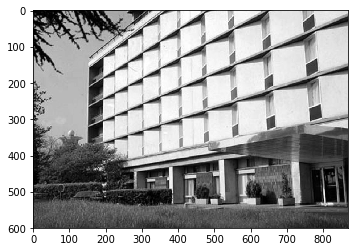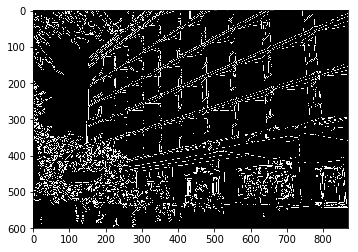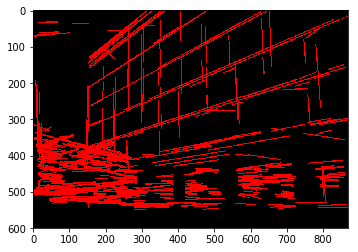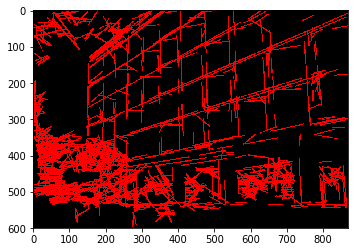## Introduction 介绍

Hough变换最初是为了识别直线（Line）而开发的，并且后来被广义化为涵盖任意形状。

## Hough space and Hough Transform 霍夫空间与霍夫变换

### 直线在 Hough space 中的表示

\begin{equation} y=a·x+b \tag{1} \end{equation}

$b=-x·a+y$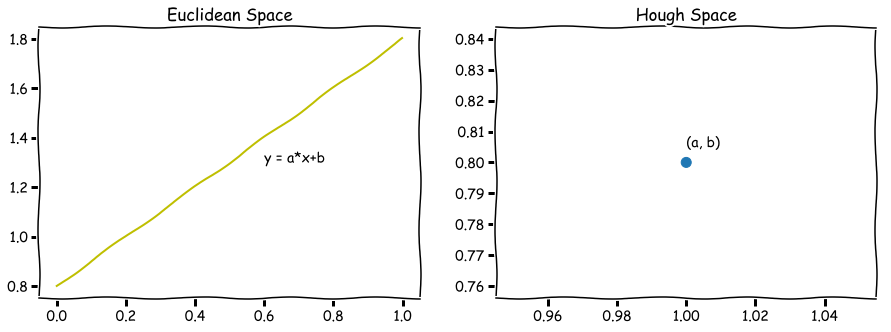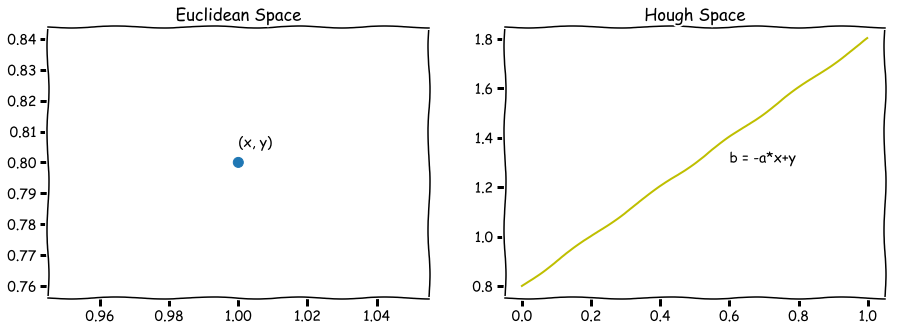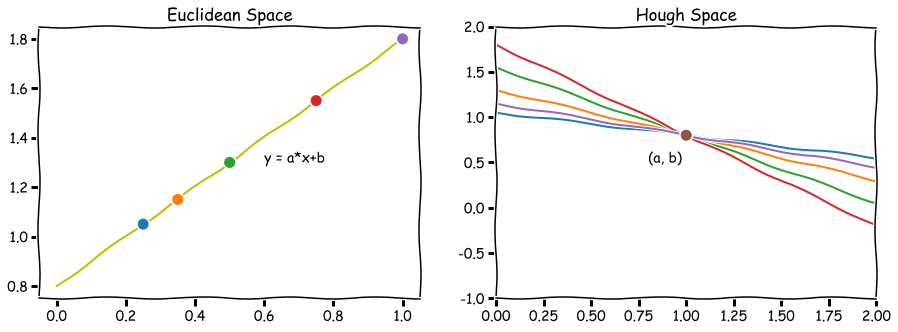### 极坐标系下

\begin{equation} r=x·cos\theta + y·sin\theta \tag{2} \end{equation}

\begin{equation} y=-\frac{cos\theta}{sin\theta}·x+\frac{r}{sin\theta} \tag{3} \end{equation}

$\theta \in [0, 180]$$r \in \mathbf{R}$（或者$\theta \in [0, 360]$$r\geqslant 0$）时，极坐标系中所有的直线都可以用这种形式表示。

Hough空间因此具有这两个维度: $\theta$$r$，并且极坐标系下直线由Hough空间的单个点表示，对应于唯一的一组参数（$\theta_0$$r_0$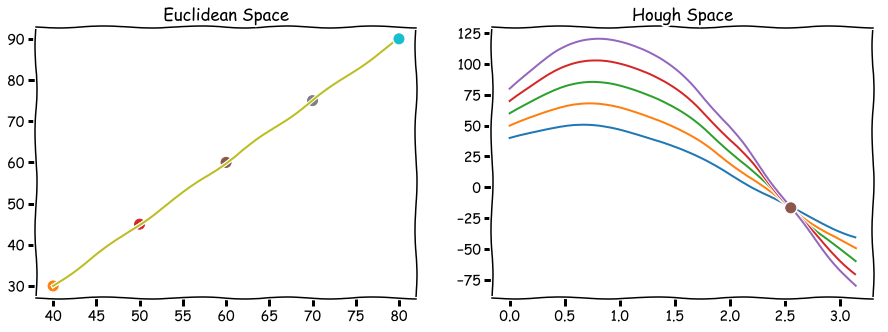## Algorithm 算法

### Detection of Infinite Lines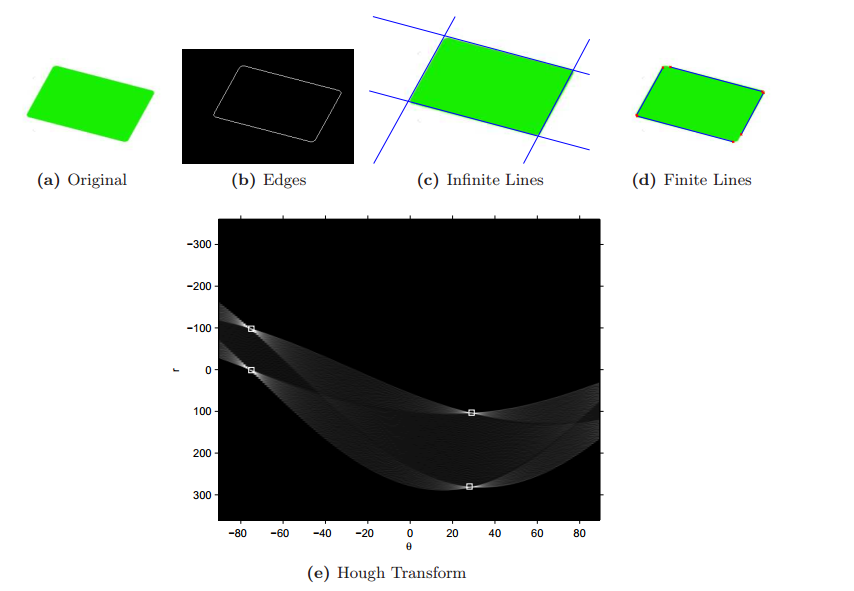### Finite line

• 一种方法是存储累加器中所有点的坐标信息，并使用这些信息来限制线条。 但是，这会导致累加器使用更多的内存。
• 另一种方法是沿Edge图像中无限长的直线搜索以找到有限长度的直线。 这种方法的一种变体称为 Progressive Probabilistic Hough Transform（渐进概率霍夫变换）

## Progressive Probabilistic Hough Transform 渐进概率霍夫变换

1. 创建输入边 Edge 图像（IMG1）的副本（IMG2）。
2. 用从 IMG2 随机选择的像素更新累加器。
3. 从 IMG2 中删除像素。
4. 如果修改的累加器（BINX）中具有最大值的较低阈值，转到第1点。
5. 沿着由BINX指定的线在 IMG1 中搜索，找到连续或间隙不超过给定阈值的间隔的最长像素段。
6. 从 IMG2 中删除段中的像素。
7. 清除BINX。
8. 如果检测到的线段比给定的最小长度长，请将其添加到输出列表中。
9. 转到第2点

## 步骤与应用

1. 边缘检测（edge detection），例如：Canny edge detection
2. 将edge point映射到霍夫空间并存储在累加器中。
3. 通过阈值和可能的其他限制，寻找累加器中最合理的点（例如，寻找累加器中值最大的一点 或者 大于阈值的点），产生无限长度的直线。
4. 将无限长度的直线转换为有限长度，并重叠在原始图像上。

Hough Transform 霍夫变换本身应用在第2点

opencv 以及 skimage 库都实现了 Hough transform 以及 ** Progressive Probabilistic Hough Transform** ，以直接调用其提供的算法接口。